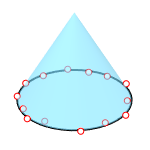# Cone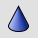Solid

Cone

The Cone command creates a surface cone.

Command-line options

### DirectionConstraint

Direction constraints restrict the direction of the cone.

##### None

Pick or type a number to set the height.##### Vertical

Creates a cone perpendicular to the construction plane.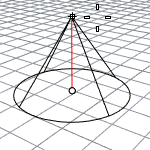• The CPlane +Z direction is the positive direction when you type a number to set the height.
##### AroundCurve

Draws the base circle perpendicular to the picked point on a curve. The center line of the cone will be tangent to the curve.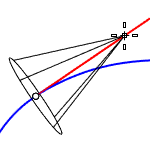• The curve direction is the positive direction when you type a number to set the height.

### Solid (Yes/No)

Decides if the bottom will be capped to create a solid or open cone.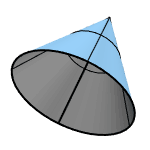Draws the base circle by picking the center point and a radius point.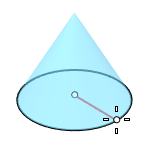### 2Point

Draws the base circle from two opposite points.### 3Point

Draws the base circle through three points.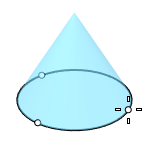### Tangent

Draws the base circle tangent to one, two, or three curves.### FitPoints

Draws the base circle by fitting to selected points, control points, or mesh vertices.